mscroggs.co.uk
mscroggs.co.uksubscribe

# Blog

2020-02-04
 This is the second post in a series of posts about my PhD thesis.
During my PhD, I spent a lot of time working on the open source boundary element method Python library Bempp. The second chapter of my thesis looks at this software, and some of the work we did to improve its performance and to make solving problems with it more simple, in more detail.

### Discrete spaces

We begin by looking at the definitions of the discrete function spaces that we will use when performing discretisation. Imagine that the boundary of our region has been split into a mesh of triangles. (The pictures in this post show a flat mesh of triangles, although in reality this mesh will usually be curved.)
We define the discrete spaces by defining a basis function of the space. The discrete space will have one of these basis functions for each triangle, for each edge, or for each vertex (or a combination of these) and the space is defined to contain all the sums of multiples of these basis functions.
The first space we define is DP0 (discontinuous polynomials of degree 0). A basis function of this space has the value 1 inside one triangle, and has the value 0 elsewhere; it looks like this:
Next we define the P1 (continuous polynomials of degree 1) space. A basis function of this space has the value 1 at one vertex in the mesh, 0 at every other vertex, and is linear inside each triangle; it looks like this:
Higher degree polynomial spaces can be defined, but we do not use them here.
For Maxwell's equations, we need different basis functions, as the unknowns are vector functions. The two most commonly spaces are RT (Raviart–Thomas) and NC (Nédélec) spaces. Example basis functions of these spaces look like this:RT (left) and NC (right) basis functions.

### Preconditioning

Suppose we are trying to solve $$\mathbf{A}\mathbf{x}=\mathbf{b}$$, where $$\mathbf{A}$$ is a matrix, $$\mathbf{b}$$ is a (known) vector, and $$\mathbf{x}$$ is the vector we are trying to find. When $$\mathbf{A}$$ is a very large matrix, it is common to only solve this approximately, and many methods are known that can achieve good approximations of the solution. To get a good idea of how quickly these methods will work, we can calculate the condition number of the matrix: the condition number is a value that is big when the matrix will be slow to solve (we call the matrix ill-conditioned); and is small when the matrix will be fast to solve (we call the matrix well-conditioned).
The matrices we get when using the boundary element method are often ill-conditioned. To speed up the solving process, it is common to use preconditioning: instead of solving $$\mathbf{A}\mathbf{x}=\mathbf{b}$$, we can instead pick a matrix $$\mathbf{P}$$ and solve $$\mathbf{P}\mathbf{A}\mathbf{x}=\mathbf{P}\mathbf{b}.$$ If we choose the matrix $$\mathbf{P}$$ carefully, we can obtain a matrix $$\mathbf{P}\mathbf{A}$$ that has a lower condition number than $$\mathbf{A}$$, so this new system could be quicker to solve.
When using the boundary element method, it is common to use properties of the Calderón projector to work out some good preconditioners. For example, the single layer operator $$\mathsf{V}$$ when discretised is often ill-conditioned, but the product of it and the hypersingular operator $$\mathsf{W}\mathsf{V}$$ is often better conditioned. This type of preconditioning is called operator preconditioning or Calderón preconditioning.
If the product $$\mathsf{W}\mathsf{V}$$ is discretised, the result is $$\mathbf{W}\mathbf{M}^{-1}\mathbf{V},$$ where $$\mathbf{W}$$ and $$\mathbf{V}$$ are discretisations of $$\mathsf{W}$$ and $$\mathsf{V}$$, and $$\mathbf{M}$$ is a matrix called the mass matrix that depends on the discretisation spaces used to discretise $$\mathsf{W}$$ and $$\mathsf{V}$$.
In our software Bempp, the mass matrices $$\mathbf{M}$$ are automatically included in product like this, which makes using preconditioning like this easier to program.
As an alternative to operator preconditioning, a method called mass matrix preconditioning is often used: this method uses the inverse mass matrix $$\mathbf{M}^{-1}$$ as a preconditioner (so is like the operator preconditioning example without the $$\mathbf{W}$$).

### More discrete spaces

As the inverse mass matrix $$\mathbf{M}^{-1}$$ appears everywhere in the preconditioning methods we would like to use, it would be great if this matrix was well-conditioned: as if it is, it's inverse can be very quickly and accurately approximated.
There is a condition called the inf-sup condition: if the inf-sup condition holds for the discretisation spaces used, then the mass matrix will be well-conditioned. Unfortunately, the inf-sup condition does not hold when using a combination of DP0 and P1 spaces.
All is not lost, however, as there are spaces we can use that do satisfy the inf-sup condition. We call these DUAL0 and DUAL1, and they form inf-sup stable pairs with P1 and DP0 (respectively). They are defined using the barycentric dual mesh: this mesh is defined by joining each point in a triangle with the midpoint of the opposite side, then making polygons with all the small triangles that touch a vertex in the original mesh: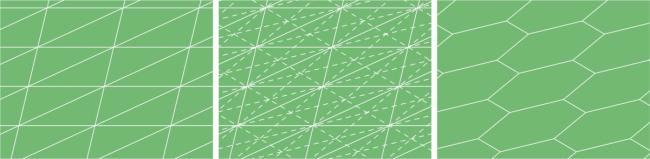The mesh (left), the barycentric refinement (centre), and the dual grid (right)
Example DUAL1 and DUAL0 basis functions look like this: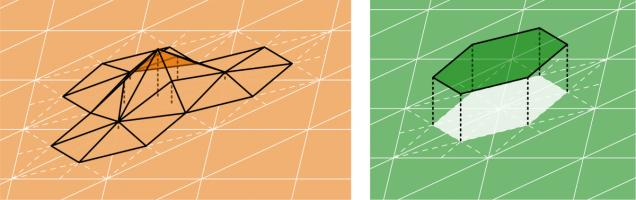DUAL1 (left) and DUAL0 (right) basis functions.
For Maxwell's equations, we define BC (Buffa–Christiansen) and RBC (rotated BC) functions to make inf-sup stable spaces pairs. Example BC and RBC basis functions look like this: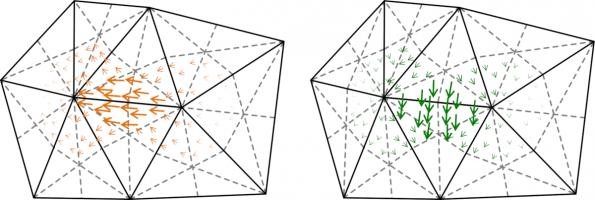Example BC (left) and RBC (right) basis functions.

My thesis then gives some example Python scripts that show how these spaces can be used in Bempp to solve some example problems, concluding chapter 2 of my thesis. Why not take a break and have a slice of the following figure before reading on.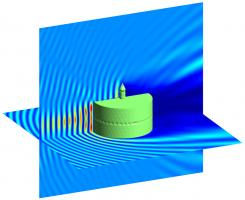An electromagnetic wave scattering off a perfectly conducting metal cake. This solution was found using a Calderón preconditioned boundary element method.
Previous post in seriesPhD thesis, chapter 1
This is the second post in a series of posts about my PhD thesis.
Next post in series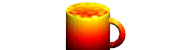PhD thesis, chapter 3

### Similar postsPhD thesis, chapter ∞PhD thesis, chapter 5PhD thesis, chapter 4PhD thesis, chapter 3

Comments in green were written by me. Comments in blue were not written by me.

Allowed HTML tags: <br> <a> <small> <b> <i> <s> <sup> <sub> <u> <spoiler> <ul> <ol> <li>
To prove you are not a spam bot, please type "k" then "i" then "t" then "e" in the box below (case sensitive):
2017-03-08
This post appeared in issue 05 of Chalkdust. I strongly recommend reading the rest of Chalkdust.
Take a long strip of paper. Fold it in half in the same direction a few times. Unfold it and look at the shape the edge of the paper makes. If you folded the paper $$n$$ times, then the edge will make an order $$n$$ dragon curve, so called because it faintly resembles a dragon. Each of the curves shown on the cover of issue 05 of Chalkdust is an order 10 dragon curve.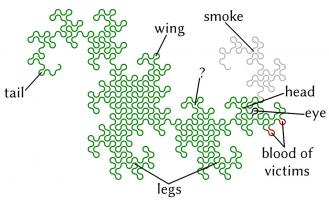Top: Folding a strip of paper in half four times leads to an order four dragon curve (after rounding the corners). Bottom: A level 10 dragon curve resembling a dragon.
The dragon curves on the cover show that it is possible to tile the entire plane with copies of dragon curves of the same order. If any readers are looking for an excellent way to tile a bathroom, I recommend getting some dragon curve-shaped tiles made.
An order $$n$$ dragon curve can be made by joining two order $$n-1$$ dragon curves with a 90° angle between their tails. Therefore, by taking the cover's tiling of the plane with order 10 dragon curves, we may join them into pairs to get a tiling with order 11 dragon curves. We could repeat this to get tilings with order 12, 13, and so on... If we were to repeat this ad infinitum we would arrive at the conclusion that an order $$\infty$$ dragon curve will cover the entire plane without crossing itself. In other words, an order $$\infty$$ dragon curve is a space-filling curve.
Like so many other interesting bits of recreational maths, dragon curves were popularised by Martin Gardner in one of his Mathematical Games columns in Scientific American. In this column, it was noted that the endpoints of dragon curves of different orders (all starting at the same point) lie on a logarithmic spiral. This can be seen in the diagram below.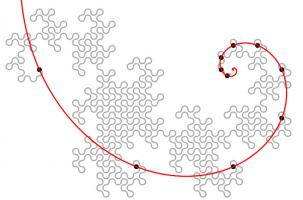The endpoints of dragon curves of order 1 to 10 with a logarithmic spiral passing through them.
Although many of their properties have been known for a long time and are well studied, dragon curves continue to appear in new and interesting places. At last year's Maths Jam conference, Paul Taylor gave a talk about my favourite surprise occurrence of a dragon.
Normally when we write numbers, we write them in base ten, with the digits in the number representing (from right to left) ones, tens, hundreds, thousands, etc. Many readers will be familiar with binary numbers (base two), where the powers of two are used in the place of powers of ten, so the digits represent ones, twos, fours, eights, etc.
In his talk, Paul suggested looking at numbers in base -1+i (where i is the square root of -1; you can find more adventures of i here) using the digits 0 and 1. From right to left, the columns of numbers in this base have values 1, -1+i, -2i, 2+2i, -4, etc. The first 11 numbers in this base are shown below.
 Number in base -1+i Complex number 0 0 1 1 10 -1+i 11 (-1+i)+(1)=i 100 -2i 101 (-2i)+(1)=1-2i 110 (-2i)+(-1+i)=-1-i 111 (-2i)+(-1+i)+(1)=-i 1000 2+2i 1001 (2+2i)+(1)=3+2i 1010 (2+2i)+(-1+i)=1+3i
Complex numbers are often drawn on an Argand diagram: the real part of the number is plotted on the horizontal axis and the imaginary part on the vertical axis. The diagram to the left shows the numbers of ten digits or less in base -1+i on an Argand diagram. The points form an order 10 dragon curve! In fact, plotting numbers of $$n$$ digits or less will draw an order $$n$$ dragon curve.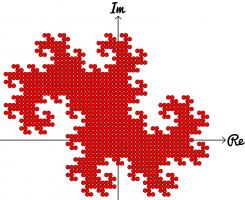Numbers in base -1+i of ten digits or less plotted on an Argand diagram.
Brilliantly, we may now use known properties of dragon curves to discover properties of base -1+i. A level $$\infty$$ dragon curve covers the entire plane without intersecting itself: therefore every Gaussian integer (a number of the form $$a+\text{i} b$$ where $$a$$ and $$b$$ are integers) has a unique representation in base -1+i. The endpoints of dragon curves lie on a logarithmic spiral: therefore numbers of the form $$(-1+\text{i})^n$$, where $$n$$ is an integer, lie on a logarithmic spiral in the complex plane.
If you'd like to play with some dragon curves, you can download the Python code used to make the pictures here.

### Similar posts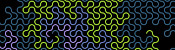Dragon curvesHarriss and other spirals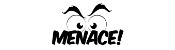MENACE at Manchester Science Festival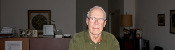The Mathematical Games of Martin Gardner

Comments in green were written by me. Comments in blue were not written by me.

Allowed HTML tags: <br> <a> <small> <b> <i> <s> <sup> <sub> <u> <spoiler> <ul> <ol> <li>
To prove you are not a spam bot, please type "naidem" backwards in the box below (case sensitive):
2016-10-08
During my Electromagnetic Field talk this year, I spoke about @mathslogicbot, my Twitter bot that is working its way through the tautologies in propositional calculus. My talk included my conjecture that the number of tautologies of length $$n$$ is an increasing sequence (except when $$n=8$$). After my talk, Henry Segerman suggested that I also look at the number of contradictions of length $$n$$ to look for insights.
A contradiction is the opposite of a tautology: it is a formula that is False for every assignment of truth values to the variables. For example, here are a few contradictions:
$$\neg(a\leftrightarrow a)$$ $$\neg(a\rightarrow a)$$ $$(\neg a\wedge a)$$ $$(\neg a\leftrightarrow a)$$
The first eleven terms of the sequence whose $$n$$th term is the number of contradictions of length $$n$$ are:
$$0, 0, 0, 0, 0, 6, 2, 20, 6, 127, 154$$
This sequence is A277275 on OEIS. A list of contractions can be found here.
For the same reasons as the sequence of tautologies, I would expect this sequence to be increasing. Surprisingly, it is not increasing for small values of $$n$$, but I again conjecture that it is increasing after a certain point.

### Properties of the sequences

There are some properties of the two sequences that we can show. Let $$a(n)$$ be the number of tautolgies of length $$n$$ and let $$b(n)$$ be the number of contradictions of length $$n$$.
First, the number of tautologies and contradictions, $$a(n)+b(n)$$, (A277276) is an increasing sequence. This is due to the facts that $$a(n+1)\geq b(n)$$ and $$b(n+1)\geq a(n)$$, as every tautology of length $$n$$ becomes a contraction of length $$n+1$$ by appending a $$\neg$$ to be start and vice versa.
This implies that for each $$n$$, at most one of $$a$$ and $$b$$ can be decreasing at $$n$$, as if both were decreasing, then $$a+b$$ would be decreasing. Sadly, this doesn't seem to give us a way to prove the conjectures, but it is a small amount of progress towards them.

### Similar posts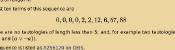Logic bot, pt. 2Logic botInteresting tautologies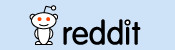How OEISbot works

Comments in green were written by me. Comments in blue were not written by me.

Allowed HTML tags: <br> <a> <small> <b> <i> <s> <sup> <sub> <u> <spoiler> <ul> <ol> <li>
To prove you are not a spam bot, please type "htdiw" backwards in the box below (case sensitive):
2016-10-06
Thanks to Marc, I noticed that today's date is a palindrome in two different date formats—DMMYY (61016) and DMMYYYY (6102016).
This made me wonder when there will be another date that is palindromic in multiple date formats, so I wrote a Python script to find out.
Turns out there's not too long to wait: 10 July 2017 will be palindromic in two date formats (MDDYY and MDDYYYY). But before that, there's 1 July 2017, which is palindromic in three date formats (YYMMD, YYMD and MDYY). Most exciting of all, however, is 2 February 2020, which is palindromic in 7 different formats!
The next palindromic dates are shown in the following table. It will update as the dates pass.
 $$n$$ Next day with $$\geq n$$ palindromic formats Formats 1 12 August 2021 YYMDD,DDMYY 2 12 August 2021 YYMDD,DDMYY 3 2 December 2021 YYYYMMDD,MMDDYYYY,MMDYY 4 2 February 2022 YYYYMDD,YYMMD,YYMD,DMYY,MDYY 5 2 February 2022 YYYYMDD,YYMMD,YYMD,DMYY,MDYY 6 1 January 2211 YYMMD,DMYYYY,MDYYYY,YYMD,DMYY,MDYY
A full list of future palindromic dates can be found here.

### Similar postsPhD thesis, chapter 2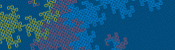Dragon curves IILogical contradictionsDragon curves

Comments in green were written by me. Comments in blue were not written by me.

Allowed HTML tags: <br> <a> <small> <b> <i> <s> <sup> <sub> <u> <spoiler> <ul> <ol> <li>
To prove you are not a spam bot, please type "k" then "i" then "t" then "e" in the box below (case sensitive):
2016-03-30
Take a piece of paper. Fold it in half in the same direction many times. Now unfold it. What pattern will the folds make?
I first found this question in one of Martin Gardner's books. At first, you might that the answer will be simple, but if you look at the shapes made for a few folds, you will see otherwise:
The curves formed are called dragon curves as they allegedly look like dragons with smoke rising from their nostrils. I'm not sure I see the resemblance:
As you increase the order of the curve (the number of times the paper was folded), the dragon curve squiggles across more of the plane, while never crossing itself. In fact, if the process was continued forever, an order infinity dragon curve would cover the whole plane, never crossing itself.
This is not the only way to cover a plane with dragon curves: the curves tessellate.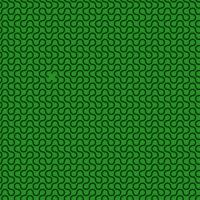When tiled, this picture demonstrates how dragon curves tessellate. For a demonstration, try obtaining infinite lives...
Dragon curves of different orders can also fit together:
To generate digital dragon curves, first notice that an order $$n$$ curve can be made from two order $$n-1$$ curves:
This can easily be seen to be true if you consider folding paper: If you fold a strip of paper in half once, then $$n-1$$ times, each half of the strip will have made an order $$n-1$$ dragon curve. But the whole strip has been folded $$n$$ times, so is an order $$n$$ dragon curve.
Because of this, higher order dragons can be thought of as lots of lower order dragons tiled together. An the infinite dragon curve is actually equivalent to tiling the plane with a infinite number of dragons.
If you would like to create your own dragon curves, you can download the Python code I used to draw them from GitHub. If you are more of a thinker, then you might like to ponder what difference it would make if the folds used to make the dragon were in different directions.

### Similar postsDragon curves IIPhD thesis, chapter 2Visualising MENACE's learningHarriss and other spirals

Comments in green were written by me. Comments in blue were not written by me.